In my previous session of Geometry, I discussed some properties of triangles. Also, I shared some important questions for SSC CGL. Today I'm going to discuss congruency and similarity of triangles which are one of the most important properties of triangles. There are some rules to determine whether the given two triangles are congruent or similar or nothing. Lets discuss it one by one.

## Congruency of Triangles

Two triangles are said to congruent if
• they overlap each other when placed one over the other.
• they have same area.
• identical in all aspects.

### When two triangles are congruent?

Now, the question is how we can detect that whether the given two triangles are congruent or not.

There are 5 rules or I can say ways by which we decide whether the triangles are congruent are not.

#### 1. S-S-S (Side- Side-Side)

When two given triangles have same three sides,then both are said to be congruent triangle.

(MARKED WITH BLUE COLOR to EQUAL TERMS.)For example, following two triangles have equal sides. Therefore, these are congruent triangles#### 2. S-A-S (Side- Angle- Side)

When two sides and included angle is same (A is in between two S's), then also both triangles are congruent.Following two triangles are congruent:#### 3. A-S-A (Angle- Side- Angle)

When two angles and included side of both triangles are equal (S is in between two A), then triangles are congruent.Following two triangles are similar: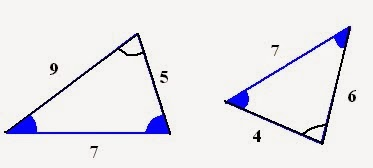#### 4. A-A-S (Angle- Angle- Side)

When two angles and non-included side (  not the included side) is equal, then both triangles are congruent.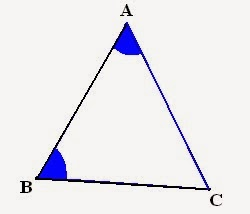Following two triangles are congruent:#### 5. R-H-S ( Right angle- Hypotenuse- Side)

This rule is just for right angle triangles. For congruency, there must be one right angle and hypotenuse and any one side must be equal.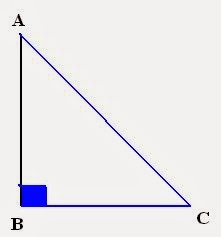Following two right angle triangles are congruent: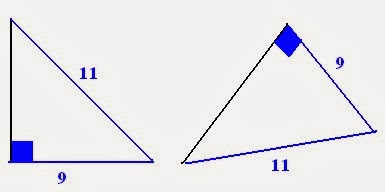### Special Case:

#### A-A-A (Angle- Angle- Angle)

Two triangle having all three same angles are NOT congruent because you cant assume that they will have same sides i.e. same angles does not mean sides will be same.

## Similarity of triangles

Two triangles are said to be similar if
• sides of both triangles are in same proportion.
• corresponding angle are same
• different size and area

### When two triangles are Similar?

To check whether two triangles are similar or not, there are only 3 rules. Discussed as follows:

#### 1. A-A ( Angle- Angle)

If any two angles of triangles are equal, then they are said to be similar. For example, following triangles are similar triangles: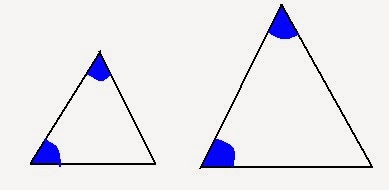#### 2. S-A-S (Side- Angle- Side)

If two side of triangles are proportionate and include angle is same, then both triangles are similar.
Consider the following triangle:
7/3.5 = 10/5 =2 i.e. proportionate sides. Also one angle is same. Therefore, these are similar triangles.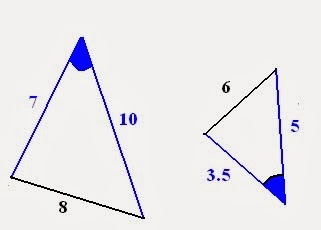#### 3. S-S-S (Side- Side- Side)

If three sides of one triangle is proportionate to three side of other triangle, then they are said to be similar triangle.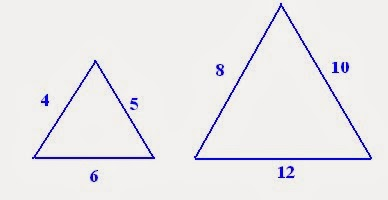All the three sides are proportional.

8/4 = (12)/6 = (10)/5 =2
Therefore, triangles are similar.

Remember that,
All congruent triangles are similar. But, all similar triangles are not congruent.

Geometry (Part-2) for SSC CGL Tier-IReviewed by Jasleen Behl on Sunday, February 23, 2014 Rating: 5